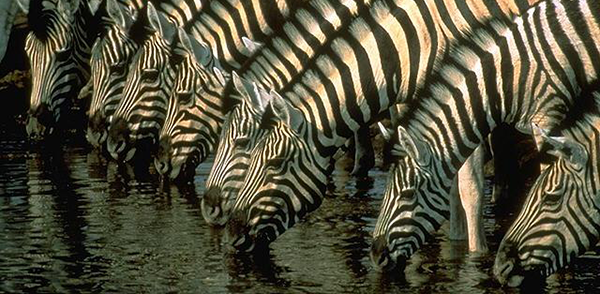# Interesting MultiplesFor how many positive integers n where $1 \le n \le 1000$ does there exist some multiple of n which is a sequence of 1s followed by a sequence of 0s in base 10?

$\textbf{ Details and Examples }$

There must be at least one 1 at the beginning of the multiple and it must end in at least one 0

10 is such an n since it itself satisfies the conditions

6 is such an n since $6 \times 185 = 1110$ which also satisfies the conditions

Image credit: Zebra Photo Gallery

×